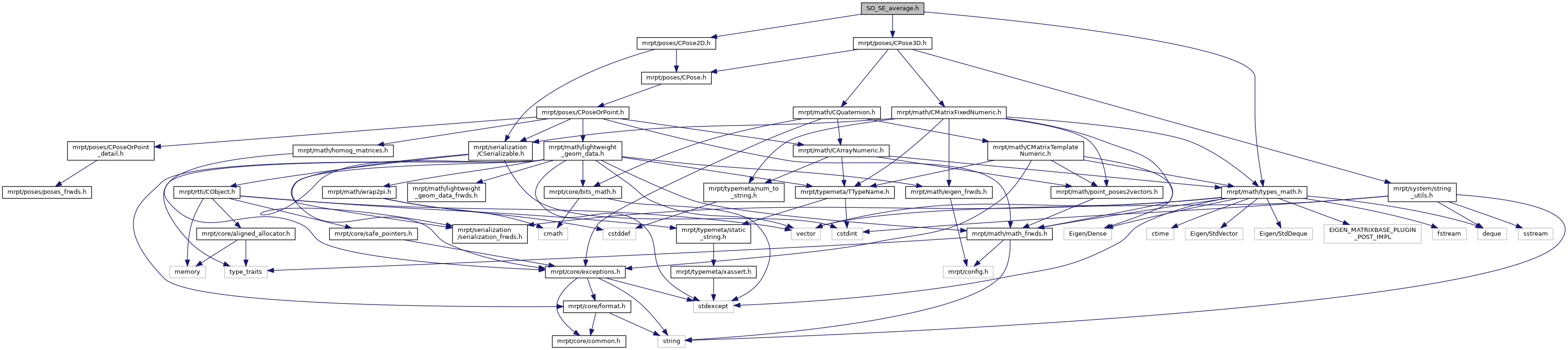MRPT  1.9.9
SO_SE_average.h File Reference
`#include <mrpt/poses/CPose3D.h>`
`#include <mrpt/poses/CPose2D.h>`
`#include <mrpt/math/types_math.h>`
Include dependency graph for SO_SE_average.h:This graph shows which files directly or indirectly include this file:Go to the source code of this file.

## Classes

class  mrpt::poses::SO_average< DOF >
Computes weighted and un-weighted averages of SO(2) or SO(3) orientations. More...

class  mrpt::poses::SE_average< DOF >
Computes weighted and un-weighted averages of SE(2) or SE(3) poses. More...

class  mrpt::poses::SO_average< 2 >
Computes weighted and un-weighted averages of SO(2) orientations. More...

class  mrpt::poses::SO_average< 3 >
Computes weighted and un-weighted averages of SO(3) orientations. More...

class  mrpt::poses::SE_average< 2 >
Computes weighted and un-weighted averages of SE(2) poses. More...

class  mrpt::poses::SE_average< 3 >
Computes weighted and un-weighted averages of SE(3) poses. More...

## Namespaces

mrpt::poses
Classes for 2D/3D geometry representation, both of single values and probability density distributions (PDFs) in many forms.

 Page generated by Doxygen 1.8.14 for MRPT 1.9.9 Git: 7d5e6d718 Fri Aug 24 01:51:28 2018 +0200 at lun nov 2 08:35:50 CET 2020